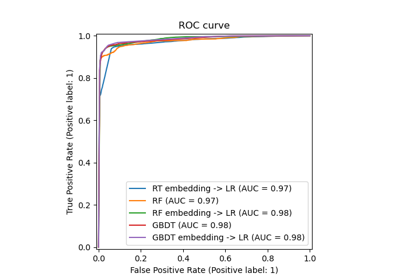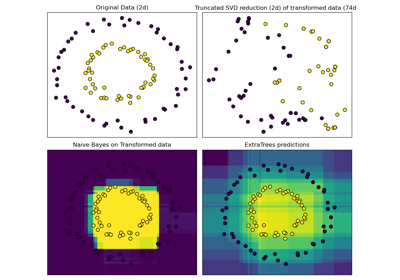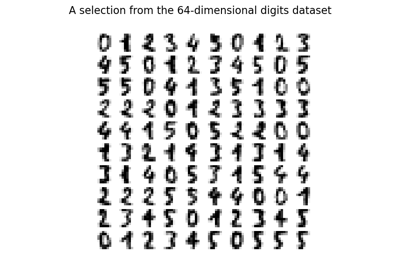# sklearn.ensemble.RandomTreesEmbedding¶

class sklearn.ensemble.RandomTreesEmbedding(n_estimators=100, *, max_depth=5, min_samples_split=2, min_samples_leaf=1, min_weight_fraction_leaf=0.0, max_leaf_nodes=None, min_impurity_decrease=0.0, sparse_output=True, n_jobs=None, random_state=None, verbose=0, warm_start=False)[source]

An ensemble of totally random trees.

An unsupervised transformation of a dataset to a high-dimensional sparse representation. A datapoint is coded according to which leaf of each tree it is sorted into. Using a one-hot encoding of the leaves, this leads to a binary coding with as many ones as there are trees in the forest.

The dimensionality of the resulting representation is n_out <= n_estimators * max_leaf_nodes. If max_leaf_nodes == None, the number of leaf nodes is at most n_estimators * 2 ** max_depth.

Read more in the User Guide.

Parameters:
n_estimatorsint, default=100

Number of trees in the forest.

Changed in version 0.22: The default value of n_estimators changed from 10 to 100 in 0.22.

max_depthint, default=5

The maximum depth of each tree. If None, then nodes are expanded until all leaves are pure or until all leaves contain less than min_samples_split samples.

min_samples_splitint or float, default=2

The minimum number of samples required to split an internal node:

• If int, then consider min_samples_split as the minimum number.

• If float, then min_samples_split is a fraction and ceil(min_samples_split * n_samples) is the minimum number of samples for each split.

Changed in version 0.18: Added float values for fractions.

min_samples_leafint or float, default=1

The minimum number of samples required to be at a leaf node. A split point at any depth will only be considered if it leaves at least min_samples_leaf training samples in each of the left and right branches. This may have the effect of smoothing the model, especially in regression.

• If int, then consider min_samples_leaf as the minimum number.

• If float, then min_samples_leaf is a fraction and ceil(min_samples_leaf * n_samples) is the minimum number of samples for each node.

Changed in version 0.18: Added float values for fractions.

min_weight_fraction_leaffloat, default=0.0

The minimum weighted fraction of the sum total of weights (of all the input samples) required to be at a leaf node. Samples have equal weight when sample_weight is not provided.

max_leaf_nodesint, default=None

Grow trees with max_leaf_nodes in best-first fashion. Best nodes are defined as relative reduction in impurity. If None then unlimited number of leaf nodes.

min_impurity_decreasefloat, default=0.0

A node will be split if this split induces a decrease of the impurity greater than or equal to this value.

The weighted impurity decrease equation is the following:

N_t / N * (impurity - N_t_R / N_t * right_impurity
- N_t_L / N_t * left_impurity)


where N is the total number of samples, N_t is the number of samples at the current node, N_t_L is the number of samples in the left child, and N_t_R is the number of samples in the right child.

N, N_t, N_t_R and N_t_L all refer to the weighted sum, if sample_weight is passed.

New in version 0.19.

sparse_outputbool, default=True

Whether or not to return a sparse CSR matrix, as default behavior, or to return a dense array compatible with dense pipeline operators.

n_jobsint, default=None

The number of jobs to run in parallel. fit, transform, decision_path and apply are all parallelized over the trees. None means 1 unless in a joblib.parallel_backend context. -1 means using all processors. See Glossary for more details.

random_stateint, RandomState instance or None, default=None

Controls the generation of the random y used to fit the trees and the draw of the splits for each feature at the trees’ nodes. See Glossary for details.

verboseint, default=0

Controls the verbosity when fitting and predicting.

warm_startbool, default=False

When set to True, reuse the solution of the previous call to fit and add more estimators to the ensemble, otherwise, just fit a whole new forest. See Glossary and Fitting additional weak-learners for details.

Attributes:
estimator_ExtraTreeRegressor instance

The child estimator template used to create the collection of fitted sub-estimators.

New in version 1.2: base_estimator_ was renamed to estimator_.

base_estimator_ExtraTreeRegressor instance

Estimator used to grow the ensemble.

estimators_list of ExtraTreeRegressor instances

The collection of fitted sub-estimators.

feature_importances_ndarray of shape (n_features,)

The impurity-based feature importances.

n_features_in_int

Number of features seen during fit.

New in version 0.24.

feature_names_in_ndarray of shape (n_features_in_,)

Names of features seen during fit. Defined only when X has feature names that are all strings.

New in version 1.0.

n_outputs_int

The number of outputs when fit is performed.

one_hot_encoder_OneHotEncoder instance

One-hot encoder used to create the sparse embedding.

ExtraTreesClassifier

An extra-trees classifier.

ExtraTreesRegressor

An extra-trees regressor.

RandomForestClassifier

A random forest classifier.

RandomForestRegressor

A random forest regressor.

sklearn.tree.ExtraTreeClassifier

An extremely randomized tree classifier.

sklearn.tree.ExtraTreeRegressor

An extremely randomized tree regressor.

References



P. Geurts, D. Ernst., and L. Wehenkel, “Extremely randomized trees”, Machine Learning, 63(1), 3-42, 2006.



Moosmann, F. and Triggs, B. and Jurie, F. “Fast discriminative visual codebooks using randomized clustering forests” NIPS 2007

Examples

>>> from sklearn.ensemble import RandomTreesEmbedding
>>> X = [[0,0], [1,0], [0,1], [-1,0], [0,-1]]
>>> random_trees = RandomTreesEmbedding(
...    n_estimators=5, random_state=0, max_depth=1).fit(X)
>>> X_sparse_embedding = random_trees.transform(X)
>>> X_sparse_embedding.toarray()
array([[0., 1., 1., 0., 1., 0., 0., 1., 1., 0.],
[0., 1., 1., 0., 1., 0., 0., 1., 1., 0.],
[0., 1., 0., 1., 0., 1., 0., 1., 0., 1.],
[1., 0., 1., 0., 1., 0., 1., 0., 1., 0.],
[0., 1., 1., 0., 1., 0., 0., 1., 1., 0.]])


Methods

 Apply trees in the forest to X, return leaf indices. Return the decision path in the forest. fit(X[, y, sample_weight]) Fit estimator. fit_transform(X[, y, sample_weight]) Fit estimator and transform dataset. get_feature_names_out([input_features]) Get output feature names for transformation. Get metadata routing of this object. get_params([deep]) Get parameters for this estimator. set_fit_request(*[, sample_weight]) Request metadata passed to the fit method. set_output(*[, transform]) Set output container. set_params(**params) Set the parameters of this estimator. Transform dataset.
apply(X)[source]

Apply trees in the forest to X, return leaf indices.

Parameters:
X{array-like, sparse matrix} of shape (n_samples, n_features)

The input samples. Internally, its dtype will be converted to dtype=np.float32. If a sparse matrix is provided, it will be converted into a sparse csr_matrix.

Returns:
X_leavesndarray of shape (n_samples, n_estimators)

For each datapoint x in X and for each tree in the forest, return the index of the leaf x ends up in.

property base_estimator_

Estimator used to grow the ensemble.

decision_path(X)[source]

Return the decision path in the forest.

New in version 0.18.

Parameters:
X{array-like, sparse matrix} of shape (n_samples, n_features)

The input samples. Internally, its dtype will be converted to dtype=np.float32. If a sparse matrix is provided, it will be converted into a sparse csr_matrix.

Returns:
indicatorsparse matrix of shape (n_samples, n_nodes)

Return a node indicator matrix where non zero elements indicates that the samples goes through the nodes. The matrix is of CSR format.

n_nodes_ptrndarray of shape (n_estimators + 1,)

The columns from indicator[n_nodes_ptr[i]:n_nodes_ptr[i+1]] gives the indicator value for the i-th estimator.

property feature_importances_

The impurity-based feature importances.

The higher, the more important the feature. The importance of a feature is computed as the (normalized) total reduction of the criterion brought by that feature. It is also known as the Gini importance.

Warning: impurity-based feature importances can be misleading for high cardinality features (many unique values). See sklearn.inspection.permutation_importance as an alternative.

Returns:
feature_importances_ndarray of shape (n_features,)

The values of this array sum to 1, unless all trees are single node trees consisting of only the root node, in which case it will be an array of zeros.

fit(X, y=None, sample_weight=None)[source]

Fit estimator.

Parameters:
X{array-like, sparse matrix} of shape (n_samples, n_features)

The input samples. Use dtype=np.float32 for maximum efficiency. Sparse matrices are also supported, use sparse csc_matrix for maximum efficiency.

yIgnored

Not used, present for API consistency by convention.

sample_weightarray-like of shape (n_samples,), default=None

Sample weights. If None, then samples are equally weighted. Splits that would create child nodes with net zero or negative weight are ignored while searching for a split in each node. In the case of classification, splits are also ignored if they would result in any single class carrying a negative weight in either child node.

Returns:
selfobject

Returns the instance itself.

fit_transform(X, y=None, sample_weight=None)[source]

Fit estimator and transform dataset.

Parameters:
X{array-like, sparse matrix} of shape (n_samples, n_features)

Input data used to build forests. Use dtype=np.float32 for maximum efficiency.

yIgnored

Not used, present for API consistency by convention.

sample_weightarray-like of shape (n_samples,), default=None

Sample weights. If None, then samples are equally weighted. Splits that would create child nodes with net zero or negative weight are ignored while searching for a split in each node. In the case of classification, splits are also ignored if they would result in any single class carrying a negative weight in either child node.

Returns:
X_transformedsparse matrix of shape (n_samples, n_out)

Transformed dataset.

get_feature_names_out(input_features=None)[source]

Get output feature names for transformation.

Parameters:
input_featuresarray-like of str or None, default=None

Only used to validate feature names with the names seen in fit.

Returns:
feature_names_outndarray of str objects

Transformed feature names, in the format of randomtreesembedding_{tree}_{leaf}, where tree is the tree used to generate the leaf and leaf is the index of a leaf node in that tree. Note that the node indexing scheme is used to index both nodes with children (split nodes) and leaf nodes. Only the latter can be present as output features. As a consequence, there are missing indices in the output feature names.

Get metadata routing of this object.

Please check User Guide on how the routing mechanism works.

Returns:

A MetadataRequest encapsulating routing information.

get_params(deep=True)[source]

Get parameters for this estimator.

Parameters:
deepbool, default=True

If True, will return the parameters for this estimator and contained subobjects that are estimators.

Returns:
paramsdict

Parameter names mapped to their values.

set_fit_request(*, sample_weight: Union[bool, None, str] = '$UNCHANGED$') [source]

Request metadata passed to the fit method.

Note that this method is only relevant if enable_metadata_routing=True (see sklearn.set_config). Please see User Guide on how the routing mechanism works.

The options for each parameter are:

• True: metadata is requested, and passed to fit if provided. The request is ignored if metadata is not provided.

• False: metadata is not requested and the meta-estimator will not pass it to fit.

• None: metadata is not requested, and the meta-estimator will raise an error if the user provides it.

• str: metadata should be passed to the meta-estimator with this given alias instead of the original name.

The default (sklearn.utils.metadata_routing.UNCHANGED) retains the existing request. This allows you to change the request for some parameters and not others.

New in version 1.3.

Note

This method is only relevant if this estimator is used as a sub-estimator of a meta-estimator, e.g. used inside a Pipeline. Otherwise it has no effect.

Parameters:
sample_weightstr, True, False, or None, default=sklearn.utils.metadata_routing.UNCHANGED

Metadata routing for sample_weight parameter in fit.

Returns:
selfobject

The updated object.

set_output(*, transform=None)[source]

Set output container.

See Introducing the set_output API for an example on how to use the API.

Parameters:
transform{“default”, “pandas”}, default=None

Configure output of transform and fit_transform.

• "default": Default output format of a transformer

• "pandas": DataFrame output

• None: Transform configuration is unchanged

Returns:
selfestimator instance

Estimator instance.

set_params(**params)[source]

Set the parameters of this estimator.

The method works on simple estimators as well as on nested objects (such as Pipeline). The latter have parameters of the form <component>__<parameter> so that it’s possible to update each component of a nested object.

Parameters:
**paramsdict

Estimator parameters.

Returns:
selfestimator instance

Estimator instance.

transform(X)[source]

Transform dataset.

Parameters:
X{array-like, sparse matrix} of shape (n_samples, n_features)

Input data to be transformed. Use dtype=np.float32 for maximum efficiency. Sparse matrices are also supported, use sparse csr_matrix for maximum efficiency.

Returns:
X_transformedsparse matrix of shape (n_samples, n_out)

Transformed dataset.

## Examples using sklearn.ensemble.RandomTreesEmbedding¶Feature transformations with ensembles of trees

Feature transformations with ensembles of treesHashing feature transformation using Totally Random Trees

Hashing feature transformation using Totally Random TreesManifold learning on handwritten digits: Locally Linear Embedding, Isomap…

Manifold learning on handwritten digits: Locally Linear Embedding, Isomap...